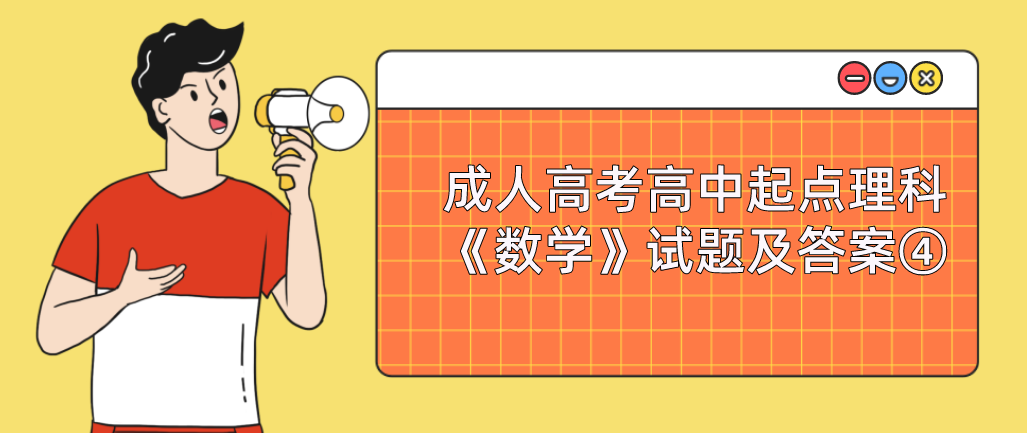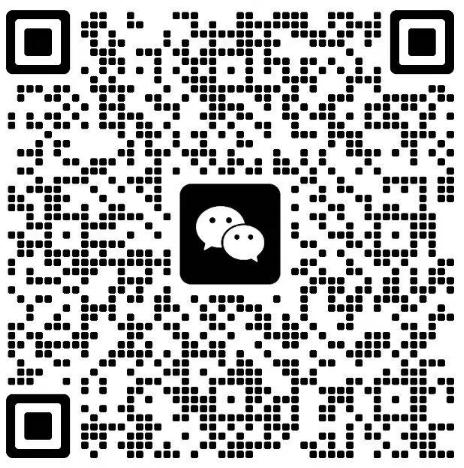# 2022年浙江成人高考高中起点理科《数学》试题及答案④18.已知a=(1,2),b=(-2,3),则(a+b)·(a-b)=()

a+b=(1,2)+(-2,3)=(-1,5),

a-b=(1.2)-(-2.3)=(3,-1).

19.曲线y=x+1在点(1,2)处的切线方程是()

y=3x2.yl.1=3,故曲线在点(1,2)处的切线方程为3(x-1)=y-2,

20.从某班的一次数学测验试卷中取出10张作为一个样本，记录试卷的得分为86、91、100、72、93、89、90、85、75、95

21.已知i，j，k是彼此互相垂直的单位向量，向量a=3i-2j+k，b=4i+3j-6k，则a·b=()

22.在9与243中间插入两个数，使它们同这两个数成等比数列，那么这两个数为()、()。

2023年全国成人高考还有

### 浙江成考网在线客服